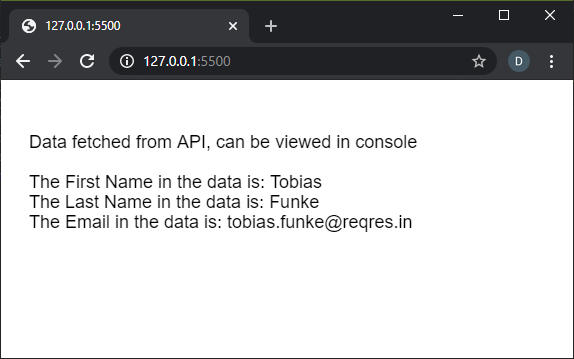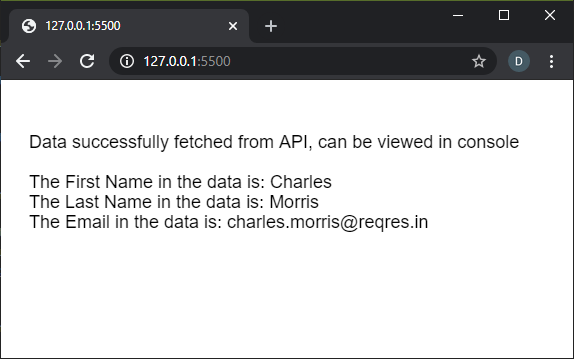Open in App
Not now

# p5.js | httpGet() Function

• Last Updated : 13 Dec, 2021

The httpGet() function in p5.js is used to execute an HTTP GET request. The datatype returned is automatically guessed by p5 based on the URL, when it is not specified.
The data could be loaded in the preload() function so that it can be accessed immediately in the program.
Syntax:

`httpGet( path, [datatype], [data], [callback], [errorCallback] )`

OR

`httpGet( path, data, [callback], [errorCallback] )`

OR

`httpGet( path, callback, [errorCallback] )`

Parameters: This function accepts five parameters as mentioned above and described below.

• path: It is a string that denotes the path of the URL or file to load.
• datatype: It is a string that specifies the type of data that will be received. It can have the values of “json”, “jsonp”, “binary”, “arrayBuffer”, “xml”, or “text”. Using the ‘binary’ datatype will return a Blob object and using ‘arrayBuffer’ will return a typed array. It will default to ‘text’ is no parameter is specified. It is an optional parameter.
• data: It is an Object or a Boolean value that specifies the parameter data passed with the request.
• callback: It is a function which is called when this function executes successfully. The first argument for this function is the data returned from the API. It is an optional parameter.
• errorCallback: It is a function which is called if there is any error in executing the function. The first argument for this function is the error response. It is an optional parameter.

Return Value: It returns a promise that can be resolved with the data when the operation completes successfully, or be rejected when an error takes place.
Below examples illustrate the httpGet() function in p5.js:
Example 1:

## javascript

 `let user_data;` `function` `preload() {` `  ``// Get a random user from the test API``  ``let api_url =``    ``'https://reqres.in/api/users/'` `+ int(random(1, 10));` `  ``httpGet(api_url, ``'json'``, ``false``, ``function` `(response) {``    ``user_data = response;``  ``});` `  ``// Log the received data to console``  ``console.log(user_data);``}` `function` `setup() {``  ``createCanvas(550, 200);``  ``textSize(18);``}` `function` `draw() {``  ``clear();``  ``if` `(!user_data)``    ``return``;` `  ``text("Data fetched from API, can be viewed "``        ``+ "``in` `console", 20, 60);` `  ``text("The First Name ``in` `the data is: "``       ``+ user_data.data.first_name, 20, 100);` `  ``text("The Last Name ``in` `the data is: "``       ``+ user_data.data.last_name, 20, 120);` `  ``text("The Email ``in` `the data is: "``       ``+ user_data.data.email, 20, 140);``}`

Output:Example 2:

## javascript

 `function` `setup() {``  ``createCanvas(550, 200);``  ``textSize(18);` `  ``// Get a random user from the test API``  ``let api_url =``    ``'https://reqres.in/api/users/'` `+ int(random(1, 10));` `  ``httpGet(api_url, ``'json'``, ``false``, onSuccessfulFetch, onErrorFetch);``}` `function` `onSuccessfulFetch(response) {``  ``text("Data successfully fetched from API, "``      ``+ "can be viewed ``in` `console", 20, 60);` `  ``text("The First Name ``in` `the data is: "``      ``+ response.data.first_name, 20, 100);` `  ``text("The Last Name ``in` `the data is: "``      ``+ response.data.last_name, 20, 120);` `  ``text("The Email ``in` `the data is: "``      ``+ response.data.email, 20, 140);``}` `function` `onErrorFetch() {``  ``text("There was an error fetching the data.", 20, 60);``}`

Output:Online editor: https://editor.p5js.org/
Environment Setup: https://www.geeksforgeeks.org/p5-js-soundfile-object-installation-and-methods/
Reference: https://p5js.org/reference/#/p5/httpGet

My Personal Notes arrow_drop_up